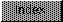```

NAME
ScalerDiv -- Get the scaling result that BitMapScale would. (V36)

SYNOPSIS
result = ScalerDiv(factor, numerator, denominator)
D0                 D0      D1         D2

UWORD ScalerDiv(UWORD, UWORD, UWORD);

FUNCTION
Calculate the expression (factor*numerator/denominator) such
that the result is the same as the width of the destination
result of BitMapScale when the factor here is the width of
the source, and the numerator and denominator are the
XDestFactor and XSrcFactor for BitMapScale.

INPUTS
factor                 - a number in the range 0..16383
numerator, denominator - numbers in the range 1..16383

RESULT
this returns factor*numerator/denominator

```